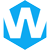# Resistor Color Code CalculatorResistor color codes are an essential aspect of working with electronic circuits. These color codes provide crucial information about the resistor’s value, tolerance, and temperature coefficient. With a variety of resistor types available, understanding the color code system is vital for professionals and hobbyists alike. This comprehensive guide aims to help you decode resistor color codes and use them effectively in your projects.

## Resistor Color Code Calculator

A resistor color code calculator is a useful tool for reading and decoding resistor color codes. By selecting the appropriate colors for each band, the calculator will display the resistor value, tolerance, and temperature coefficient (if applicable). This can be a convenient way to determine the resistor’s properties without manually consulting a color code chart.

## Introduction to Resistors

Resistors are fundamental electronic components used to restrict the flow of electric current in a circuit. They come in various forms, including fixed resistors, variable resistors, and surface-mount resistors. Fixed resistors have a single value, while variable resistors can provide different resistance values. The most common fixed resistors are carbon-composition resistors, which have color bands for easy identification.

## Resistor Color Code System

The resistor color code system is an internationally recognized standard defined by IEC 60062. This system uses different colors to represent the significant digits, multiplier, tolerance, and temperature coefficient of a resistor. Depending on the type of resistor, it may have between three and six color bands.

### 4-Band Resistor Color Code

The 4-band resistor color code is the most common variation. It consists of two bands for the resistance value, one for the multiplier, and one for the tolerance. The first two bands represent the significant digits, while the third band serves as the multiplier. The fourth band indicates the tolerance of the resistor.

### 5-Band Resistor Color Code

For higher precision resistors, an additional band is used to indicate a third significant digit. This results in three bands for the significant digits, one for the multiplier, and one for the tolerance. Some 5-band resistors may also have an additional band for the temperature coefficient or failure rate.

### 6-Band Resistor Color Code

High precision resistors with six bands have an additional band to specify the temperature coefficient. The first three bands indicate the significant digits, the fourth band is the multiplier, the fifth band represents the tolerance, and the sixth band shows the temperature coefficient.

## Resistor Color Code Chart

The resistor color code chart helps to decode the color bands on a resistor. Each color corresponds to a specific number, multiplier, tolerance, or temperature coefficient value. Refer to the chart below to understand the significance of each color in the resistor color code system.

Color1st, 2nd, 3rd Significant DigitsMultiplierToleranceTemperature Coefficient
Black0x1N/A250 ppm/K (U)
Brown1x10±1% (F)100 ppm/K (S)
Red2x100±2% (G)50 ppm/K (R)
Orange3x1K±3%15 ppm/K (P)
Yellow4x10K±4%25 ppm/K (Q)
Green5x100K±0.5% (D)20 ppm/K (Z)
Blue6x1M±0.25% (C)10 ppm/K (Z)
Violet7x10M±0.1% (B)5 ppm/K (M)
Grey8x100M±0.05% (L)1 ppm/K (K)
White9x1GN/AN/A
GoldN/Ax0.1±5% (J)N/A
SilverN/Ax0.01±10% (K)N/A
NoneN/AN/A±20% (M)N/A

## How to Read Resistor Color Codes

Reading resistor color codes can be simplified by following these steps:

• Identify the colored bands on the resistor’s body.
• Determine the significant digits represented by the first two or three bands (depending on the resistor type).
• Identify the multiplier band and calculate the resistance value by multiplying the significant digits by the multiplier.
• Locate the tolerance band and determine the tolerance percentage.

For 6-band resistors, identify the temperature coefficient band to understand how the resistance value changes with temperature.

## Resistor Color Code Exceptions

There are some exceptions to the standard resistor color code system:

• 5-band resistors with a 4th band of gold or silver are used in specialized or older resistors. These resistors have bands for significant digits, multiplier, tolerance, and temperature coefficient.
• High-voltage resistors may have gold and silver bands replaced with yellow and gray to prevent metal particles from contaminating the coating.
• A single black band on a resistor indicates a zero-ohm resistor, which serves as a wire link connecting traces on a printed circuit board (PCB).

## Resistor Combinations: Series and Parallel

Resistors can be connected in series or parallel combinations to achieve the desired resistance value in a circuit. The total resistance of resistors in series is the sum of their individual resistances, while the total resistance of resistors in parallel is determined by the reciprocal of the sum of their reciprocals.

## Resistance of a Conductor

The resistance of a conductor can be calculated using the formula R = L / (A × C), where R is the resistance, L is the length of the conductor, A is the cross-sectional area, and C is the conductivity of the material. This calculation is useful for determining the resistance of conductors in electrical circuits.

## Conclusion

Understanding the resistor color code system is crucial for working with electronic circuits. By using the color code chart, calculator, and the information provided in this guide, you can confidently identify and decode resistor color codes. This knowledge will enable you to select the appropriate resistors for your projects and ensure proper circuit performance.

#### Share:Aidan Taylor

I am Aidan Taylor and I have over 10 years of experience in the field of PCB Reverse Engineering, PCB design and IC Unlock.

## Recommended Post

### Need Help?

Don't hesitate - Get in touch today to find out how we can help take your project to the next level.

Scroll to Top## Start to Achieve Your PCB Project

Hello 👋
Can we help you? Chat with us on WhatsApp↓, or send an email to us: info@reversepcb.com